# WebGL着色器渲染小游戏实战

2021/10/28 18:05

## 项目起因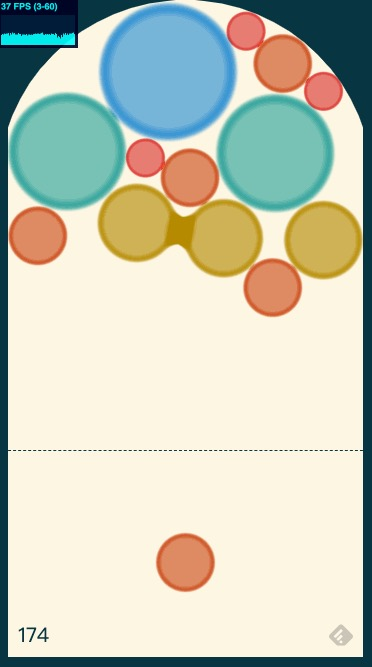## 项目结构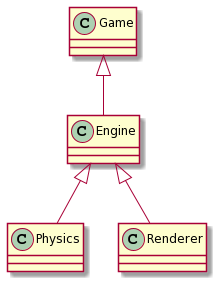## 游戏逻辑实现

### 游戏业务逻辑 Game

1. 输入HTML canvas元素，指定游戏渲染范围
2. 初始化驱动层Engine
3. 监听用户操作事件touchend/click，调用Engine控制射出泡泡
4. 循环调用Engineupdate更新方法，并检查超过指定高度的泡泡数量，如数量超过0则停止游戏
class Game {
constructor(canvas) {
this.engine = new Engine(canvas)
if(!this.isEnd) {
this.shoot({
x: e.pageX,
y: e.pageY
}, randomLevel())
}
})
}
shoot(pos, newBallLevel) {
// 已准备好的泡泡射出去
this.engine.shoot(pos, START_V)
// 在初始点生成新的泡泡
}
update() {
this.engine.update()
let point = 0;
let overflowCount = 0;
this.engine.physics.getAllBall().forEach(ball => {
if(!ball.isStatic){
point += Math.pow(2, ball.level);
if (ball.position.y > _this.sceneSize.width * 1.2) {
overflowCount++
}
}
})
if(overflowCount > 1){
this.gameEnd(point);
}
}
gameEnd(point) {
this.isEnd = true
...
}
}


### 驱动层 Engine

1. 在指定的位置生成固定的泡泡，供用户作下一次操作时使用
2. 把固定的泡泡按指定的方向射出

class Engine {
constructor(canvas) {
this.renderer = new Renderer(canvas)
this.physics = new Physics()
}
this.updateRender()
}
shoot(pos, startV) {
this.physics.shoot(pos, startV)
}
updateRender() {
// 更新渲染器渲染信息
}
update() {
// 调用渲染器更新场景渲染
this.renderer.draw()
}
}


### 物理引擎模块 Physics

1. 在指定的位置生成固定的泡泡，供用户作下一次操作时使用
2. 把固定的泡泡按指定的方向射出
3. 检查是否有相同颜色的泡泡相撞
4. 相撞的相同颜色泡泡合并为高一级的泡泡

#### 0.场景搭建

// class Physics

constructor() {
this.matterEngine = Matter.Engine.create()
// 置重力方向为y轴负方向（即为上）
this.matterEngine.world.gravity.y = -1

// 添加三面墙
...
...

// 添加上方圆顶
const path = document.getElementById('path')
const points = Matter.Svg.pathToVertices(path, 30)

Matter.Engine.run(this.matterEngine)
}


#### 1.在指定的位置生成固定的泡泡，供用户作下一次操作时使用

// class Physics

const ball = Matter.Bodies.circle(pos.x, pos.y, radius, {
...// 不同等级不同的大小通过scale区分
})
// 如果生成的是固定的泡泡，则记录在属性上供下次射出时使用
if(isStatic) {
this.stillBall = ball
}
}


#### 2.把固定的泡泡按指定的方向射出

// class Physics

// pos: 点击位置，用于计算射出方向
// startV: 射出初速度
shoot(pos, startV) {
if(this.stillBall) {
// 计算点击位置与原始位置的向量，归一化（使长度为1）之后乘以初始速度大小
let v = Matter.Vector.create(pos.x - this.stillBall.position.x, pos.y - this.stillBall.position.y)
v = Matter.Vector.normalise(v)
v = Vector.mult(v, startV)

// 设置泡泡为可活动的，并把初速度赋予泡泡
Body.setStatic(this.stillBall, false);
Body.setVelocity(this.stillBall, v);
}
}


#### 3.检查是否有相同颜色的泡泡相撞

// class Physics

checkCollision() {
// 拿到活动中的泡泡碰撞体的列表
const bodies = this.getAllBall()
let targetBody, srcBody
// 逐对泡泡碰撞体遍历
for(let i = 0; i < bodies.length; i++) {
const bodyA = bodies[i]
for(let j = i + 1; j < bodies.length; j++) {
const bodyB = bodies[j]
if(bodyA.level === bodyB.level) {
// 用距离的平方比较，避免计算开平方
// 使用靠上的泡泡作为目标泡泡
if(bodyA.position.y < bodyB.position.y) {
targetBody = bodyA
srcBody = bodyB
} else {
targetBody = bodyB
srcBody = bodyA
}
return {
srcBody,
targetBody
}
}
}
}
}
return false
}


#### 4.相撞的相同颜色泡泡合并为高一级的泡泡

1. 在每个更新周期计算源泡泡和目标泡泡位置的差值，得到源泡泡需要移动的向量
2. 移动向量的1/8，在下一个更新周期重复1、2的操作
3. 当两个泡泡的位置差值小于一个较小的值（这里设为5）时，视为合并完成，销毁源泡泡，并更新目标泡泡的等级信息
// class Physics

mergeBall(srcBody, targetBody, callback) {
const dist = Math.sqrt(getDistSq(srcBody.position, targetBody.position))
// 源泡泡位置设为固定的，且不参与碰撞
Matter.Body.setStatic(srcBody, true)
// 如果两个泡泡合并到距离小于5的时候, 目标泡泡升级为上一级的泡泡
if(dist < 5) {
// 合并后的泡泡的等级
const newLevel = Math.min(targetBody.level + 1, 8)
const scale = BallRadiusMap[newLevel] / BallRaiusMap[targetBody.level]
// 更新目标泡泡信息
Matter.Body.scale(targetBody, scale, scale)
Matter.Body.set(targetBody, {level: newLevel})
Matter.World.remove(this.matterEngine.world, srcBody)
callback()
return
}
// 需要继续播放泡泡靠近动画
const velovity = {
x: targetBody.position.x - srcBody.position.x,
y: targetBody.position.y - srcBody.position.y
};
// 泡泡移动速度先慢后快
velovity.x /= dist / 8;
velovity.y /= dist / 8;
Matter.Body.translate(srcBody, Matter.Vector.create(velovity.x, velovity.y));
}


// class Physics

constructor() {
...

Matter.Events.on(this.matterEngine, 'beforeUpdate', e => {
// 检查是否有正在合并的泡泡，没有则检测是否有相同颜色的泡泡碰撞
if(!this.collisionInfo) {
this.collisionInfo = this.checkCollision()
}
if(this.collisionInfo) {
// 若有正在合并的泡泡，（继续）调用合并方法，在合并完成后清空属性
this.mergeBall(this.collisionInfo.srcBody, this.collisionInfo.targetBody, () => {
this.collistionInfo = null
})
}
})

...
}


### 渲染器模块

GLSL渲染器的实现比较复杂，当前可以先使用matter.js自带的渲染器调试一下。

Physics模块中，再初始化一个matter.jsrender:

class Physics {
constructor(...) {
...
this.render = Matter.Render.create(...)
Matter.Render.run(this.render)
}
}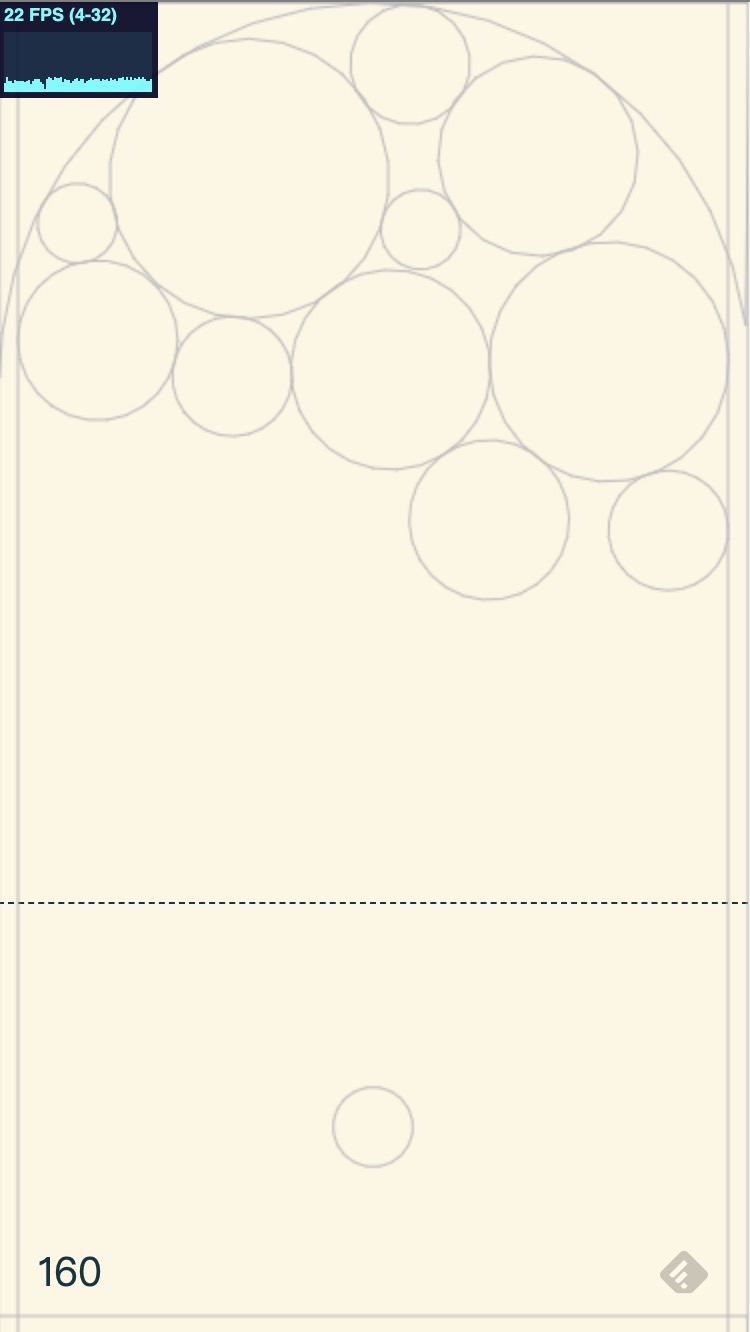## 开发定制渲染器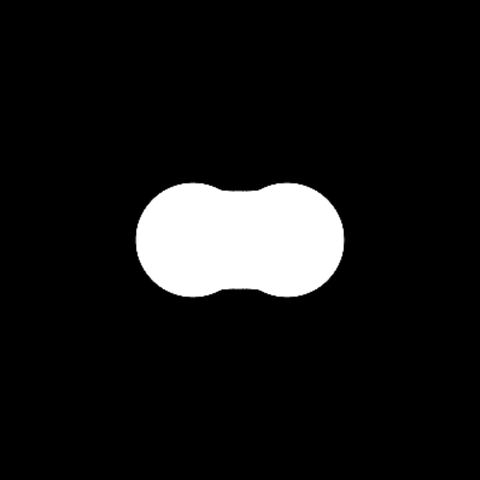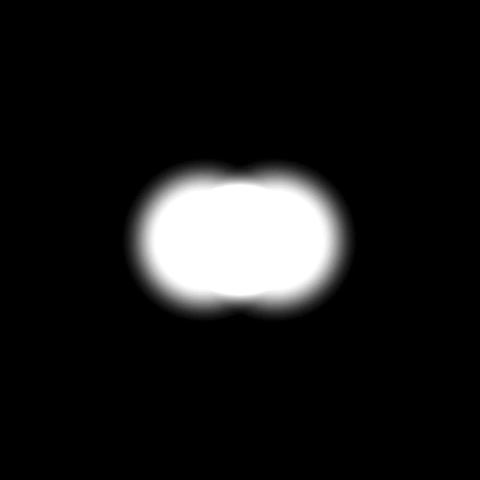## 着色器结构

// 顶点着色器 vertexShader
attribute vec2 a_Position;
attribute float a_PointSize;

void main() {
gl_Position = vec4(a_Position, 0.0, 1.0);
gl_PointSize = a_PointSize;
}

// 片段着色器 fragmentShader
#ifdef GL_ES
precision mediump float;
#endif

void main() {
float d = length(gl_PointCoord - vec2(0.5, 0.5));
float c = smoothstep(0.40, 0.20, d);
gl_FragColor = vec4(vec3(c), 1.0);
}

// 渲染器 Renderer.js
class GLRenderer {
...
// 更新游戏元素数据
updateData(posData, sizeData) {
...
this.posData = new Float32Array(posData)
this.sizeData = new Float32Array(sizeData)
...
}
// 更新渲染
draw() {
...
// 每个顶点取2个数
this.setAttribute(this.program, 'a_Position', this.posData, 2, 'FLOAT')
// 每个顶点取1个数
this.setAttribute(this.program, 'a_PointSize', this.sizeData, 1, 'FLOAT')
...
}
}


gl.blendFunc(gl.SRC_ALPHA, gl.ONE)
gl.enable(gl.BLEND);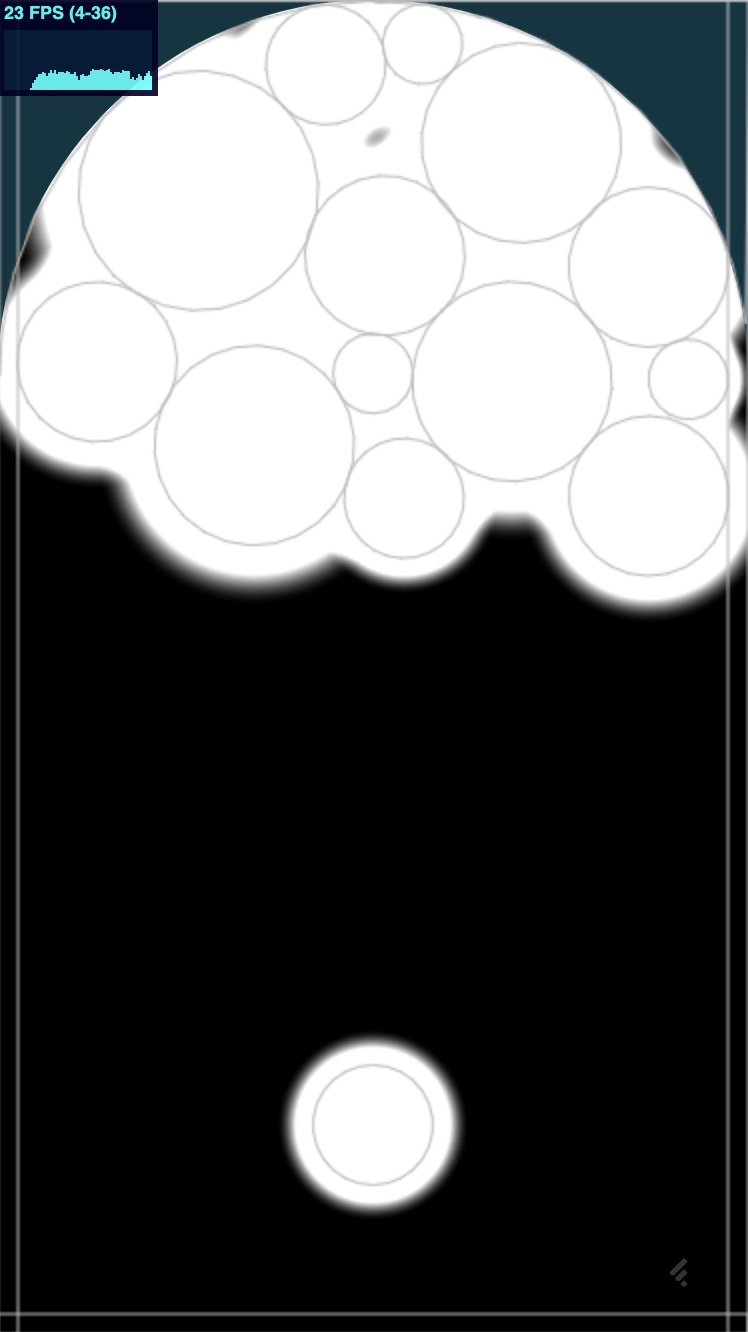// 创建frameBuffer
var frameBuffer = gl.createFramebuffer()
// 创建纹理texture
var texture = gl.createTexture()
// 绑定纹理到二维纹理
gl.bindTexture(gl.TEXTURE_2D, texture)
// 设置纹理信息，注意宽度和高度需是2的次方幂，纹理像素来源为空
gl.texImage2D(
gl.TEXTURE_2D,
0,
gl.RGBA,
1024,
1024,
0,
gl.RGBA,
gl.UNSIGNED_BYTE,
null
)
// 设置纹理缩小滤波器
gl.texParameteri(gl.TEXTURE_2D, gl.TEXTURE_MIN_FILTER, gl.LINEAR)
// frameBuffer与纹理绑定
gl.framebufferTexture2D(gl.FRAMEBUFFER, gl.COLOR_ATTACHMENT0, gl.TEXTURE_2D, texture, 0)


gl.bindFramebuffer(gl.FRAMEBUFFER, frameBuffer)


frameBuffer绘制完成，将自动存储到0号纹理中，供第二次的着色器渲染使用

// 场景顶点着色器 SceneVertexShader
attribute vec2 a_Position;
attribute vec2 a_texcoord;
varying vec2 v_texcoord;

void main() {
gl_Position = vec4(a_Position, 0.0, 1.0);
v_texcoord = a_texcoord;
}

// 场景片段着色器 SceneFragmentShader
#ifdef GL_ES
precision mediump float;
#endif

varying vec2 v_texcoord;
uniform sampler2D u_sceneMap;

void main() {
vec4 mapColor = texture2D(u_sceneMap, v_texcoord);
d = smoothstep(0.6, 0.7, mapColor.r);
gl_FragColor = vec4(vec3(d), 1.0);
}


1. a_Position: 纹理渲染的面的顶点座标，因为这里的纹理是铺满全画布，所以是画布的四个角
2. a_textcoord: 各个顶点的纹理uv座标，因为纹理大小和渲染大小不一样（纹理大小为1024*1024，渲染大小为画布大小），所以是从(0.0, 0.0)(width / 1024, height / 1024)
3. u_sceneMap: 纹理序号，用的第一个纹理，传入0
// 渲染器 Renderer.js
class Renderer {
...
drawScene() {
// 把渲染目标设回画布
gl.bindFramebuffer(gl.FRAMEBUFFER, null);
// 使用渲染场景的程序
gl.useProgram(sceneProgram);
// 设置4个顶点座标
this.setAttribute(this.sceneProgram, "a_Position", new Float32Array([
-1.0,
-1.0,

1.0,
-1.0,

-1.0,
1.0,

-1.0,
1.0,

1.0,
-1.0,

1.0,
1.0
]), 2, "FLOAT");
// 设置顶点座标的纹理uv座标
setAttribute(sceneProgram, "a_texcoord", new Float32Array([
0.0,
0.0,

canvas.width / MAPSIZE,
0.0,

0.0,
canvas.height / MAPSIZE,

0.0,
canvas.height / MAPSIZE,

canvas.width / MAPSIZE,
0.0,

canvas.width / MAPSIZE,
canvas.height / MAPSIZE
]), 2, "FLOAT");
// 设置使用0号纹理
this.setUniform1i(this.sceneProgram, 'u_sceneMap', 0);
// 用画三角形面的方法绘制
this.gl.drawArrays(this.gl.TRIANGLES, 0, 6);
}
}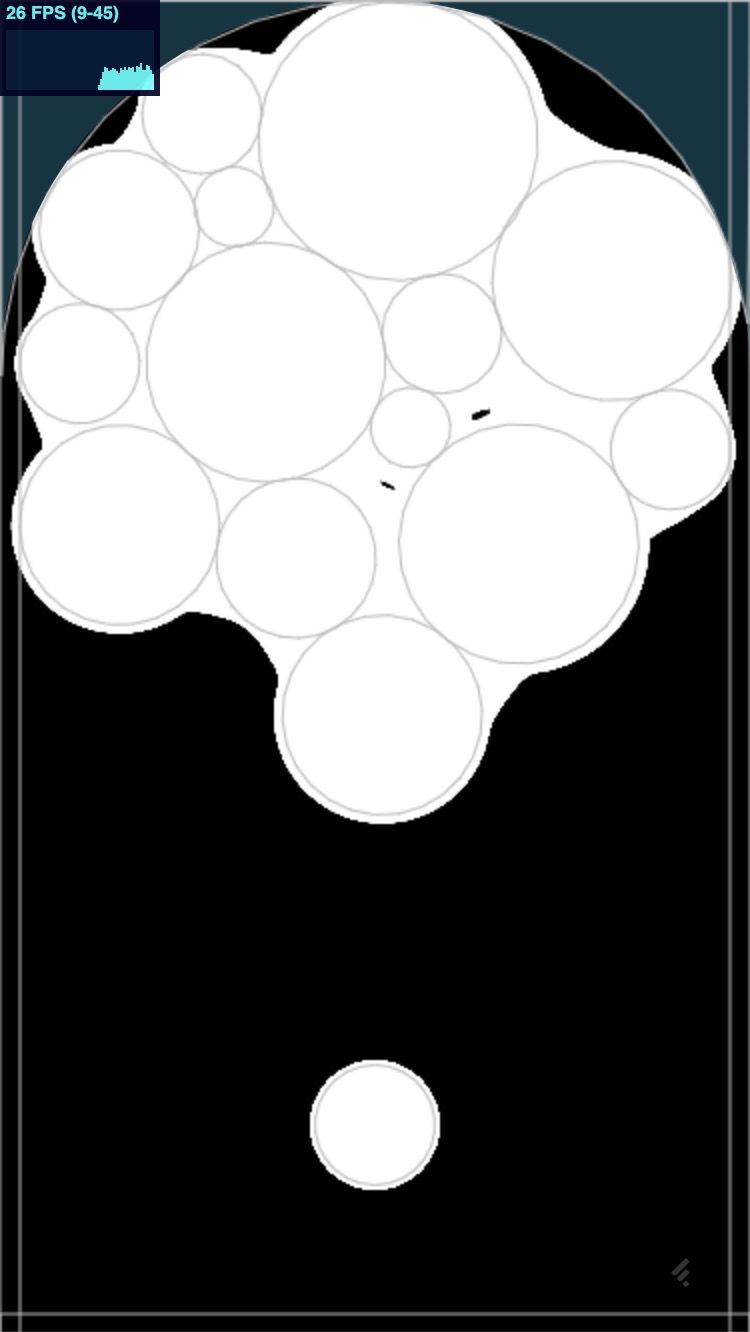## 不同类型的泡泡区别

d = smoothstep(0.6, 0.7, mapColor.r);


// 修改后的顶点着色器 vertexShader
uniform int group;// 绘制的组序号
attribute vec2 a_Position;
attribute float a_Level;// 泡泡的等级
attribute float a_PointSize;
varying vec4 v_Color;// 片段着色器该使用哪个rgb通道

void main() {
gl_Position = vec4(a_Position, 0.0, 1.0);
gl_PointSize = a_PointSize;
if(group == 0){
if(a_Level == 1.0){
v_Color = vec4(1.0, 0.0, 0.0, 1.0);// 使用r通道
}
if(a_Level == 2.0){
v_Color = vec4(0.0, 1.0, 0.0, 1.0);// 使用g通道
}
if(a_Level == 3.0){
v_Color = vec4(0.0, 0.0, 1.0, 1.0);// 使用b通道
}
}
if(group == 1){
if(a_Level == 4.0){
v_Color = vec4(1.0, 0.0, 0.0, 1.0);
}
if(a_Level == 5.0){
v_Color = vec4(0.0, 1.0, 0.0, 1.0);
}
if(a_Level == 6.0){
v_Color = vec4(0.0, 0.0, 1.0, 1.0);
}
}
if(group == 2){
if(a_Level == 7.0){
v_Color = vec4(1.0, 0.0, 0.0, 1.0);
}
if(a_Level == 8.0){
v_Color = vec4(0.0, 1.0, 0.0, 1.0);
}
if(a_Level == 9.0){
v_Color = vec4(0.0, 0.0, 1.0, 1.0);
}
}
}

// 修改后的片段着色器 fragmentShader
#ifdef GL_ES
precision mediump float;
#endif

varying vec4 v_Color;

void main(){
float d = length(gl_PointCoord - vec2(0.5, 0.5));
float c = smoothstep(0.40, 0.20, d);
gl_FragColor = v_Color * c;
}


// 修改后的场景片段着色器
#ifdef GL_ES
precision mediump float;
#endif

varying vec2 v_texcoord;
uniform sampler2D u_sceneMap;
uniform vec2 u_resolution;
uniform int group;

void main(){
vec4 mapColor = texture2D(u_sceneMap, v_texcoord);
float d = 0.0;
vec4 color = vec4(0.0);
if(group == 0){
if(mapColor.r > 0.0){
d = smoothstep(0.6, 0.7, mapColor.r);
color += vec4(0.86, 0.20, 0.18, 1.0) * d;
}
if(mapColor.g > 0.0){
d = smoothstep(0.6, 0.7, mapColor.g);
color += vec4(0.80, 0.29, 0.09, 1.0) * d;
}
if(mapColor.b > 0.0){
d = smoothstep(0.6, 0.7, mapColor.b);
color += vec4(0.71, 0.54, 0.00, 1.0) * d;
}
}
if(group == 1){
if(mapColor.r > 0.0){
d = smoothstep(0.6, 0.7, mapColor.r);
color += vec4(0.52, 0.60, 0.00, 1.0) * d;
}
if(mapColor.g > 0.0){
d = smoothstep(0.6, 0.7, mapColor.g);
color += vec4(0.16, 0.63, 0.60, 1.0) * d;
}
if(mapColor.b > 0.0){
d = smoothstep(0.6, 0.7, mapColor.b);
color += vec4(0.15, 0.55, 0.82, 1.0) * d;
}
}
if(group == 2){
if(mapColor.r > 0.0){
d = smoothstep(0.6, 0.7, mapColor.r);
color += vec4(0.42, 0.44, 0.77, 1.0) * d;
}
if(mapColor.g > 0.0){
d = smoothstep(0.6, 0.7, mapColor.g);
color += vec4(0.83, 0.21, 0.51, 1.0) * d;
}
if(mapColor.b > 0.0){
d = smoothstep(0.6, 0.7, mapColor.b);
color += vec4(1.0, 1.0, 1.0, 1.0) * d;
}
}
gl_FragColor = color;
}


// 设置WebGL每次绘制时不清空图像
var gl = canvas.getContext('webgl', {
preserveDrawingBuffer: true
});

class Renderer {
...
update() {
gl.clear(gl.COLOR_BUFFER_BIT)// 每次绘制时手动清空图像
this.drawPoint()// 绘制泡泡位置、大小
this.drawScene()// 增加阶跃滤镜
}
}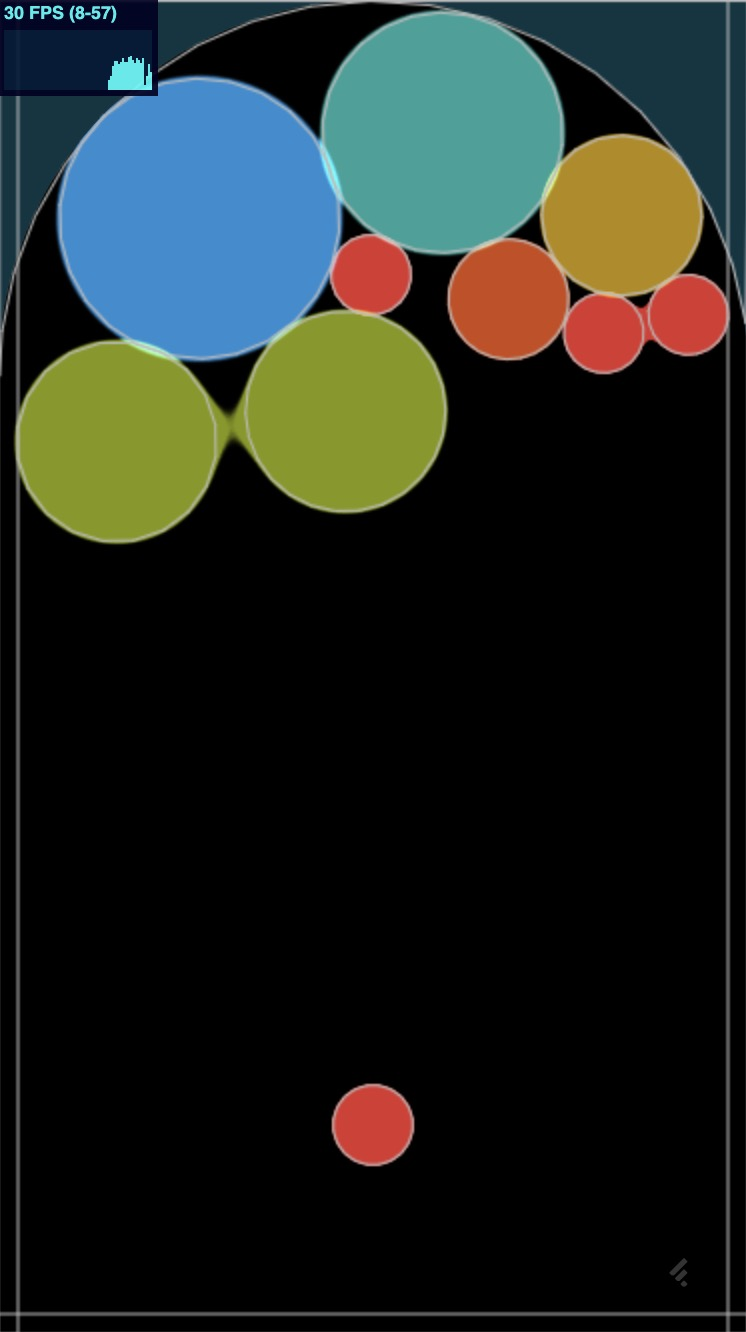## 完整项目源码可以访问: https://github.com/wenxiongid/bubble### 作者的其它热门文章

1
1 收藏

0 评论
1 收藏
1